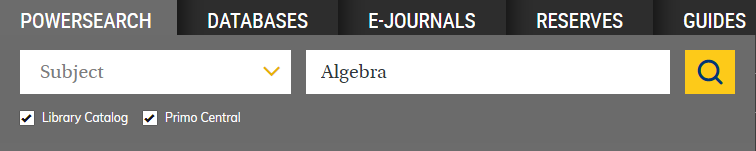# Mathematics: Reference Tools

Guide to mathematics resources

## Reference Tools

#### Dictionaries

(E-book)

(QA41.3 .Z56 2019)
The concise Oxford dictionary of mathematics
(E-book 2021)
A dictionary of statistics
(E-book QA276.14 .U67 2014)
Facts on File dictionary of mathematics
(QA5 .F35 1999)

#### Encyclopedias

MathWorld: the web's most extensive mathematics resource
(E-book 1996)
Encyclopaedia of mathematics
(E-book 1994)
Encyclopedia of mathematics and its applications
(Call numbers vary)

#### Handbooks and Tables

CRC standard mathematical tables and formulae
(E-journal 1991-)
Handbook of mathematical functions with formulas, graphs, and mathematical tables
(E-book & QA47 .H35 1972)
Mathematics handbook for science and engineering
(E-book & QA41 .R34 2004)
Table of Integrals, Series, and Products, 8th Edition
(E-book & QA55 .G6613 2000)

#### Indexes

Jahrbuch über die Fortschritte der Mathematik, 1868-1942
(E-Journal)
Statistical theory and method abstracts
(Periodical HA1 .S764)

#### Internet Resources

EMIS: the European Mathematical Information Service

The Jahrbuch Project

CTAN: the Comprehensive TeX Archive Network

Major project of digitized mathematical text:
Cornell Historical Math Monographs Project
The Electronic Library of Mathematics
University of Michigan Historical Mathematics Collection

#### Media Resources

Non-euclidean geometry: kaleidoscope, non-euclidean geometry, non-euclidean kaleidoscope (1 DVD)
(Media Resources QA3 .I577 1996a)

#### Periodicals

Search the Library Catalog using subject headings for all print and non-print materials (books, journals, magazines, newspapers, theses, electronic resources, media, microforms, etc.):• Algebra
• Computer science mathematics
• Geometry
• Mathematical statistics
• Mathematics
• Parallel processing (electronic computers)
• Probabilities
• Topology

#### Style Guides

Writing Guides and Manuals​

Guide to the successful thesis and dissertation : a handbook for students and faculty
(LB2369 .M377 2003)
Handbook of writing for the mathematical sciences
(QA42 .H54 1993)
Mathematical writing
(QA42 .K58 1989)
A primer of mathematical writing : being a disquisition on having your ideas recorded, typeset, published, read and appreciated
(E-book 2017 & QA42 .K73 1997)
Writing mathematics well : a manual for authors
(E-book QA42 .G45 1987)